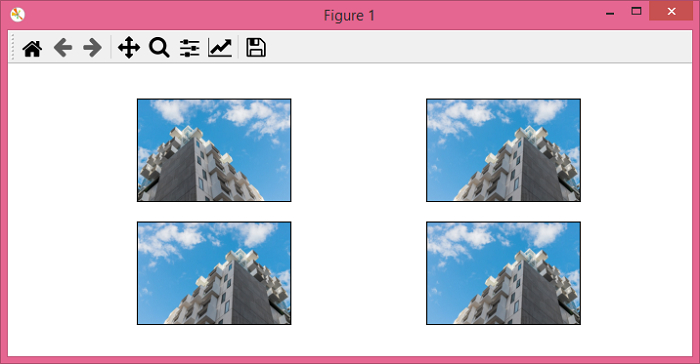# PyTorch – torchvision.transforms – RandomHorizontalFlip()

PyTorchServer Side ProgrammingProgramming

To flip an image horizontally in a random fashion with a given probability, we apply RandomHorizontalFlip() transform. It's one of the transforms provided by the torchvision.transforms module. This module contains many important transformations that can be used to perform different types of manipulations on the image data.

RandomHorizontalFlip() accepts both PIL and tensor images. A tensor image is a PyTorch Tensor with shape [C, H, W], where C is the number channels, H is the image height, and W is the image width.

## Syntax

torchvision.transforms.RandomHorizontalFlip(p)(img)
• If p = 1, it returns a horizontally flipped image.

• If p = 0, It returns the original image.

• If p is in the range (0,1), then the probability to return the horizontally flipped image is p.

It returns a horizontally flipped image randomly with a given probability p.

## Steps

We could use the following steps to horizontally flip an image randomly with a given probability −

• Import the required libraries. In all the following examples, the required Python libraries are torch, Pillow, and torchvision. Make sure you have already installed them.

import torch
import torchvision
import torchvision.transforms as T
from PIL import Image
• Read the input image. The input image is a PIL image or a tensor image.

img = Image.open('building.jpg')
• Define a transform to horizontally flip the image randomly with a given probability p. Here p = 0.25 means, the chance of any input image to be horizontally flipped is 25%.

transform = T.RandomHorizontalFlip(p = 0.25)
• Apply the above-defined transform on the input image to horizontally flip the image.

hflipped_img = transform(img)
• Show the output image.

hflipped_img.show()

## Input Image

This image is used as the input in all the following examples.## Example 1

In this program, we set p = 1, so it will definitely flip the image horizontally.

# import required libraries
import torch
import torchvision.transforms as T
from PIL import Image

# read the input image
img = Image.open('building.jpg')

# define a transform to horizontally flip an image
# randomly with a given probability
transform = T.RandomHorizontalFlip(p=1)

# apply the above defined transform on the input image
img = transform(img)

# display the image
img.show()

## Output

It will produce the following output −Notice that our output image is horizontally flipped as we set the probability p = 1.

## Example 2

In this program, we set p=0.25.

import torch
import torchvision.transforms as T
from PIL import Image
import matplotlib.pyplot as plt

img = Image.open('building.jpg')
transform = T.RandomHorizontalFlip(p=0.25)
imgs = [transform(img) for _ in range(4)]
fig = plt.figure(figsize=(7,3))
rows, cols = 2,2
for j in range(0, len(imgs)):
plt.imshow(imgs[j])
plt.xticks([])
plt.yticks([])
plt.show()

## Output

It will produce the following output −Notice that out of 4 output images, one is horizontally flipped. You may get different number of flipped images.